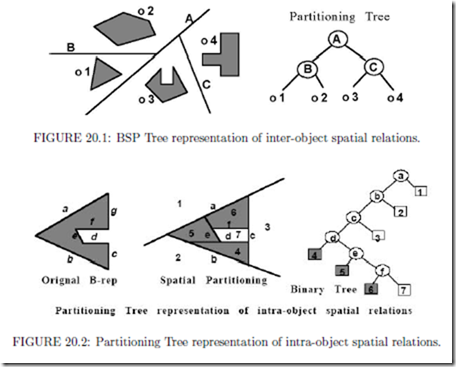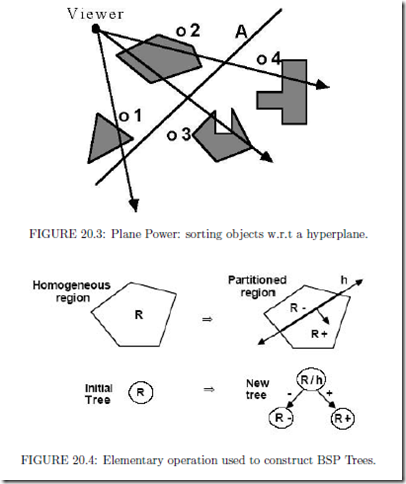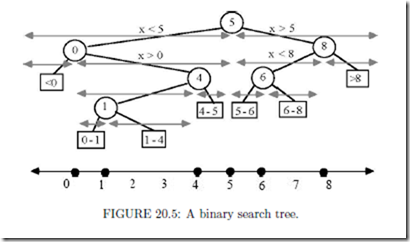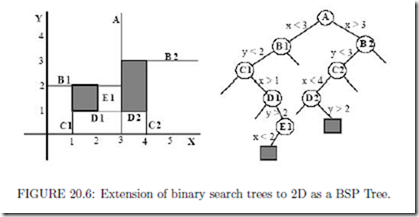# Binary Space Partitioning Trees:Introduction and Structure

###### Introduction

In most applications involving computation with 3D geometric models, manipulating objects and generating images of objects are crucial operations. Performing these operations requires determining for every frame of an animation the spatial relations between objects: how they might intersect each other, and how they may occlude each other. However, the objects, rather than being monolithic, are most often comprised of many pieces, such as by many polygons forming the faces of poly hedra. The number of pieces may be anywhere from the 100’s to the 1,000,000’s. To compute spatial relations between n polygons by brute force entails comparing every pair of polygons, and so would require O(n2). For large scenes comprised of 105 polygons, this would mean 1010 operations, which is much more than necessary.

The number of operations can be substantially reduced to anywhere from O(n log2 n) when the objects interpenetrate (and so in our example reduced to 106), to as little as constant time, O(1), when they are somewhat separated from each other. This can be accomplished by using Binary Space Partitioning Trees, also called BSP Trees. They provide a computational representation of space that simultaneously provides a search structure and a representation of geometry. The reduction in number of operations occurs because BSP Trees provide a kind of “spatial sorting”. In fact, they are a generalization to dimensions > 1 of binary search trees, which have been widely used for representing sorted lists. Figure 20.1

below gives an introductory example showing how a binary tree of lines, instead of points, can be used to “sort” four geometric objects, as opposed to sorting symbolic objects such as names.

Constructing a BSP Tree representation of one or more polyhedral objects involves computing the spatial relations between polygonal faces once and encoding these relations in a binary tree (Figure 20.2). This tree can then be transformed and merged with other trees to very quickly compute the spatial relations (for visibility and intersections) between the polygons of two moving objects.As long as the relations encoded by a tree remain valid, which for a rigid body is forever, one can reap the beneﬁts of having generated this tree structure every time the tree is used in subsequent operations. The return on investment manifests itself as substantially faster algorithms for computing intersections and visibility orderings. And for animation and interactive applications, these savings can accrue over hundreds of thousands of frames. BSP Trees achieve an elegant solution to a number of important problems in geometric computation by exploiting two very simple properties occurring whenever a single plane separates (lies between) two or more objects: 1) any object on one side of the plane cannot intersect any object on the other side, 2) given a viewing position, objects on the same side as the viewer can have their images drawn on top of the images of objects on the opposite side (Painter’s Algorithm). See Figure 20.3.

These properties can be made dimension independent if we use the term “hyper plane” to refer to planes in 3D, lines in 2D, and in general for d-space, to a (d1)-dimensional subspace deﬁned by a single linear equation. The only operation we will need for constructing BSP Trees is the partitioning of a convex region by a singe hyper plane into two child regions, both of which are also convex as a result (Figure 20.4).

BSP Trees exploit the properties of separating planes by using one very simple but powerful technique to represent any object or collection of objects: recursive subdivision by hyper planes. A BSP Tree is the recording of this process of recursive subdivision in the form of a binary tree of hyper planes. Since there is no restriction on what hyper planes are used, polytopes (polyhedra, polygons, etc.) can be represented exactly. A BSP Tree is a program for performing intersections between the hyper plane’s halfs paces and any other geometric entity. Since subdivision generates increasingly smaller regions of space, the or- der of the hyperplanes is chosen so that following a path deeper into the tree corresponds to adding more detail, yielding a multi-resolution representation. This leads to eﬃcientintersection computations. To determine visibility, all that is required is choosing at each tree node which of the two branches to draw ﬁrst based solely on which branch contains the viewer. No other single representation of geometry inherently answers questions of intersection and visibility for a scene of 3D moving objects. And this is accomplished in a computationally eﬃcient and parallelizable manner.

###### BSP Trees as a Multi-Dimensional Search Structure

Spatial search structures are based on the same ideas that were developed in Computer Science during the 60’s and 70’s to solve the problem of quickly processing large sets of symbolic data, as opposed to geometric data, such as lists of people’s names. It was dis- covered that by ﬁrst sorting a list of names alphabetically, and storing the sorted list in an array, one can ﬁnd out whether some new name is already in the list in log2 n operations using a binary search algorithm, instead of n/2 expected operations required by a sequential search. This is a good example of extracting structure (alphabetical order) existing in the list of names and exploiting that structure in subsequent operations (looking up a name) to reduce computation. However, if one wishes to permit additions and deletions of names while maintaining a sorted list, then a dynamic data structure is needed, i.e. one using pointers. One of the most common examples of such a data structure is the binary search tree.

A binary search tree (See also Chapter 3) is illustrated in Figure 20.5, where it is being used to represent a set of integers S = { 0, 1, 4, 5, 6, 8 } lying on the real line. We have included both the binary tree and the hierarchy of intervals represented by this tree. To ﬁnd out whether a number/point is already in the tree, one inserts the point into the tree and follows the path corresponding to the sequence of nested intervals that contain the point. For a balanced tree, this process will take no more than O(log n) steps; for in fact, we have performed a binary search, but one using a tree instead of an array. Indeed, the tree itself encodes a portion of the search algorithm since it prescribes the order in which the search proceeds.This now brings us back to BSP Trees, for as we said earlier, they are a generalization of binary search trees to dimensions > 1 (in 1D, they are essentially identical). In fact, constructing a BSP Tree can be thought of as a geometric version of Quick Sort. Modiﬁcations (insertions and deletions) are achieved by merging trees, analogous to merging sorted lists in Merge Sort. However, since points do not divide space for any dimension > 1, we must use hyper planes instead of points by which to subdivide. Hyper planes always partition a region into two half spaces regardless of the dimension. In 1D, they look like points since they are also 0D sets; the one diﬀerence being the addition of a normal denoting the “greater than” side. In Figure 20.6, we show a restricted variety of BSP Trees that most clearly illustrates the generalization of binary search trees to higher dimensions. (You may want to call this a k-d tree, but the standard semantics of k-d trees does not include representing continuous sets of points, but rather ﬁnite sets of points.) BSP Trees are also a geometric variety of Decision Trees, which are commonly used for classiﬁcation (e.g. biological taxonomies), and are widely used in machine learning. Decision trees have also been used for proving lower bounds, the most famous showing that sorting is Ω(n log n). They are also the model of the popular “20 questions” game (I’m thinking of something and you have 20 yes/no question to guess what it is). For BSP Trees, the questions become “what side of a particular hyper plane does some piece of geometry lie”.